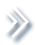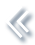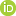####ArchivesReview Articles

## Power analysis, sample size calculation for testing the largest binomial probability

Thuan Nguyen ,

a Oregon Health and Science University, Portland, OR, USA;b University of California, Davis, CA, USAJiming Jiang

a Oregon Health and Science University, Portland, OR, USA;b University of California, Davis, CA, USAjimjiang@ucdavis.edu

Pages 78-83 | Received 31 Oct. 2018, Accepted 20 Feb. 2019, Published online: 15 Mar. 2019,
• Abstract
• References
• Citations

Abstract

A procedure is developed for power analysis and sample size calculation for a class of complex testing problems regarding the largest binomial probability under a combination of treatments. It is shown that the asymptotic null distribution of the likelihood-ratio statistic is not parameter-free, but χ21χ12 is a conservative asymptotic null distribution. A nonlinear Gauss-Seidel algorithm is proposed to uniquely determine the alternative for the power and sample size calculation given the baseline binomial probability. An example from an animal clinical trial is discussed.

## References

1. Alam, M. K., Rao, M. B., & Cheng, F.-C. (2010). Sample size determination in logistic regression. Sankhyā B72, 58–75. doi: 10.1007/s13571-010-0004-6 [Crossref], [Google Scholar]
2. Borenstein, M., Rothstein, H., & Cohen, J. (2001). Power and precision. Englewood, US: Biostat Inc. [Google Scholar]
3. Demidenko, E. (2007). Sample size determination for logistic regression revisited. Statistics in Medicine26, 3385–3397. doi: 10.1002/sim.2771 [Crossref][Web of Science ®], [Google Scholar]
4. Hsieh, F. Y., Bloch, D. A., & Larsen, M. D. (1998). A simple method of sample size calculation for linear and logistic regression. Statistics in Medicine17, 1623–1634. doi: 10.1002/(SICI)1097-0258(19980730)17:14<1623::AID-SIM871>3.0.CO;2-S [Crossref][Web of Science ®], [Google Scholar]
5. Jiang, J. (2000). A nonlinear Gauss-Seidel algorithm for inference about GLMM. Computational Statistics15, 229–241. doi: 10.1007/s001800000030 [Crossref][Web of Science ®], [Google Scholar]
6. Jiang, J. (2010). Large sample techniques for statistics. New York: Springer. [Crossref], [Google Scholar]
7. Novikov, I., Fund, N., & Freedman, L. S. (2010). A modified approach to estimating sample size for simple logistic regression with one continuous covariate. Statistics in Medicine29, 97–105. [Crossref][Web of Science ®], [Google Scholar]
8. White, H. (1982). Maximum likelihood estimation of misspecified models. Econometrica50, 1–25. doi: 10.2307/1912526 [Crossref][Web of Science ®], [Google Scholar]
9. Whittemore, A. (1981). Sample size for logistic regression with small response probability. Journal of the American Statistical Association76, 27–32. doi: 10.1080/01621459.1981.10477597 [Taylor & Francis Online][Web of Science ®], [Google Scholar]